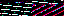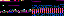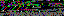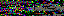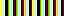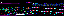```EVR.include("level/road/racer/flash/Strip.js");

{
this.racer = racer;
this.milestone_difference = FLASH_MILESTONE_DIFFERENCE;
this.active_frequency = 0;
this.last_streak = 0;
}

{
// var streak = parseInt(this.racer.level.streak);
// var difference = Math.abs(streak - this.last_streak);
// if (difference >= this.milestone_difference)
// {
//    this.setActiveLevel(streak);
//    this.displayLevel();
//    this.last_streak = streak;
// }
}

{
// var milestone = parseInt(streak/this.milestone_difference);
// if (milestone < FLASH_FREQUENCIES)
// {
//    this.active_level = milestone;
// }
}

{
// this.strip.slide(this.active_level);
}

{
// this.strip.draw();
}
```
```EVR.Level.Road.Racer.Flash.Level = function(flash, index)
{
EVR.Graphic.call(this, flash.racer.avatar);
this.flash = flash;
this.index = index;
this.animations_path = FLASH_ANIMATIONS_PATH;
this.setAttributes();
this.setImage();
this.append();
this.hide();
}
{
this.set_proportions(1, 1);
this.set_opacity(FLASH_OPACITY);
}
{
var element = document.createElement("img");
element.src = this.buildAnimationPath();
element.style.width = "100%";
element.style.height = "100%";
this.element.appendChild(element);
}
{
return this.animations_path + (this.index + 1) + ".gif";
}
{
this.css.visibility = "";
}
{
this.css.visibility = "hidden";
}
```
```<?php
\$GLOBALS["RELATIVE_IMAGE_DIRECTORY_PATH"] = "img/";
\$GLOBALS["RELATIVE_IMAGE_CACHE_PATH"] = "flash/cache/";
\$GLOBALS["IMAGE_FILE_FORMAT"] = "gif";
\$GLOBALS["FLASH_COLORS"] = array("red", "green", "blue");
\$GLOBALS["MIN_FREQUENCY"] = 10;
create_strip();
function create_strip()
{
\$height = \$_GET["height"];
\$frequencies = \$_GET["frequencies"];
\$image = build_strip(\$height, \$frequencies);
\$file_name = save_image(\$image);
echo \$file_name;
}
function build_strip(\$height, \$cell_count)
{
\$image = new Imagick();
\$min_frequency = \$GLOBALS["MIN_FREQUENCY"];
\$palette = \$GLOBALS["FLASH_COLORS"];
\$palette_count = count(\$palette);
\$frame_count = pow(2, \$cell_count - 1) * \$palette_count;
\$draw = new ImagickDraw();
for (\$frame_index = 0; \$frame_index < \$frame_count; \$frame_index++)
{
\$image->newImage(\$height * \$cell_count, \$height, "none");
\$x = 0;
for (\$cell_index = \$cell_count - 2; \$cell_index >= 0; \$cell_index--)
{
\$palette_index = (\$frame_index / pow(2, \$cell_index)) % \$palette_count;
\$color = \$palette[\$palette_index];
\$x += \$height;
\$draw->setFillColor(\$color);
\$draw->rectangle(\$x, 0, \$x + \$height, \$height);
\$image->drawImage(\$draw);
\$image->setImageDelay(\$min_frequency);
}
}
\$image->setImageFormat(\$GLOBALS["IMAGE_FILE_FORMAT"]);
return \$image;
}
function save_image(\$image)
{
go_to_save_path();
\$index = find_available_image_index();
\$file_name = build_file_name(\$index);
\$image->writeImages(\$file_name, true);
return \$file_name;
}
function go_to_save_path()
{
go_to_image_directory();
go_to_cache_directory();
}
function go_to_image_directory()
{
\$path = \$GLOBALS["RELATIVE_IMAGE_DIRECTORY_PATH"];
while (!is_dir(\$path))
{
chdir("..");
}
chdir(\$path);
}
function go_to_cache_directory()
{
\$path = \$GLOBALS["RELATIVE_IMAGE_CACHE_PATH"];
if (!is_dir(\$path))
{
\$dirs = explode("/", \$path);
foreach (\$dirs as \$dir)
{
if (\$dir != "")
{
mkdir(\$dir, 0770);
chdir(\$dir);
}
}
}
else
{
chdir(\$path);
}
}
function find_available_image_index()
{
\$index = 0;
while (count(glob(++\$index . "_*")));
return \$index;
}
function build_file_name(\$index)
{
return \$index . "_" . time() . "." . \$GLOBALS["IMAGE_FILE_FORMAT"];
}
```
```<?php
chdir("../../../../../../../../img/flash/animations/");
\$files = glob("*.gif");
echo count(\$files);
```
```EVR.Level.Road.Racer.Flash.Strip = function(flash)
{
EVR.Graphic.call(this, flash.racer.avatar);
this.index = 0;
this.step = null;
this.current_cache_index = null;
this.setAttributes();
this.append();
}
{
this.set_proportions(1, 1);
this.set_opacity(FLASH_OPACITY);
this.css.backgroundAttachment = "fixed";
}
{
this.step = -this.container.get_dimensions();
}
{
EVR.Graphic.prototype.draw.call(this);
if (this.container.get_dimensions() != -this.step)
{
this.removeCachedStrip();
this.setStep();
this.setBackground();
this.slide(this.index);
}
}
{
var index = this.current_cache_index;
if (index)
{
var script = this.buildPathToSelf() + "remove_cached_strip.php";
var query = "index=" + index;
new EVR.Requester(script, query, true).execute();
}
}
{
}
{
var script = this.buildPathToSelf() + "create_strip.php";
var query = "height=" + -this.step + "&frequencies=" + FLASH_FREQUENCIES;
var file_name = new EVR.Requester(script, query, true).execute();
var path = "img/flash/cache/" + file_name;
var cache_index = parseInt(file_name.split("_"));
this.css.background = "url('" + path + "')";
this.current_cache_index = cache_index;
}
{
this.css.backgroundPosition = index * this.step + "px 0";
this.index = index;
}
{
}
```
35.172.233.2
35.172.233.2
35.172.233.2

June 23, 2019